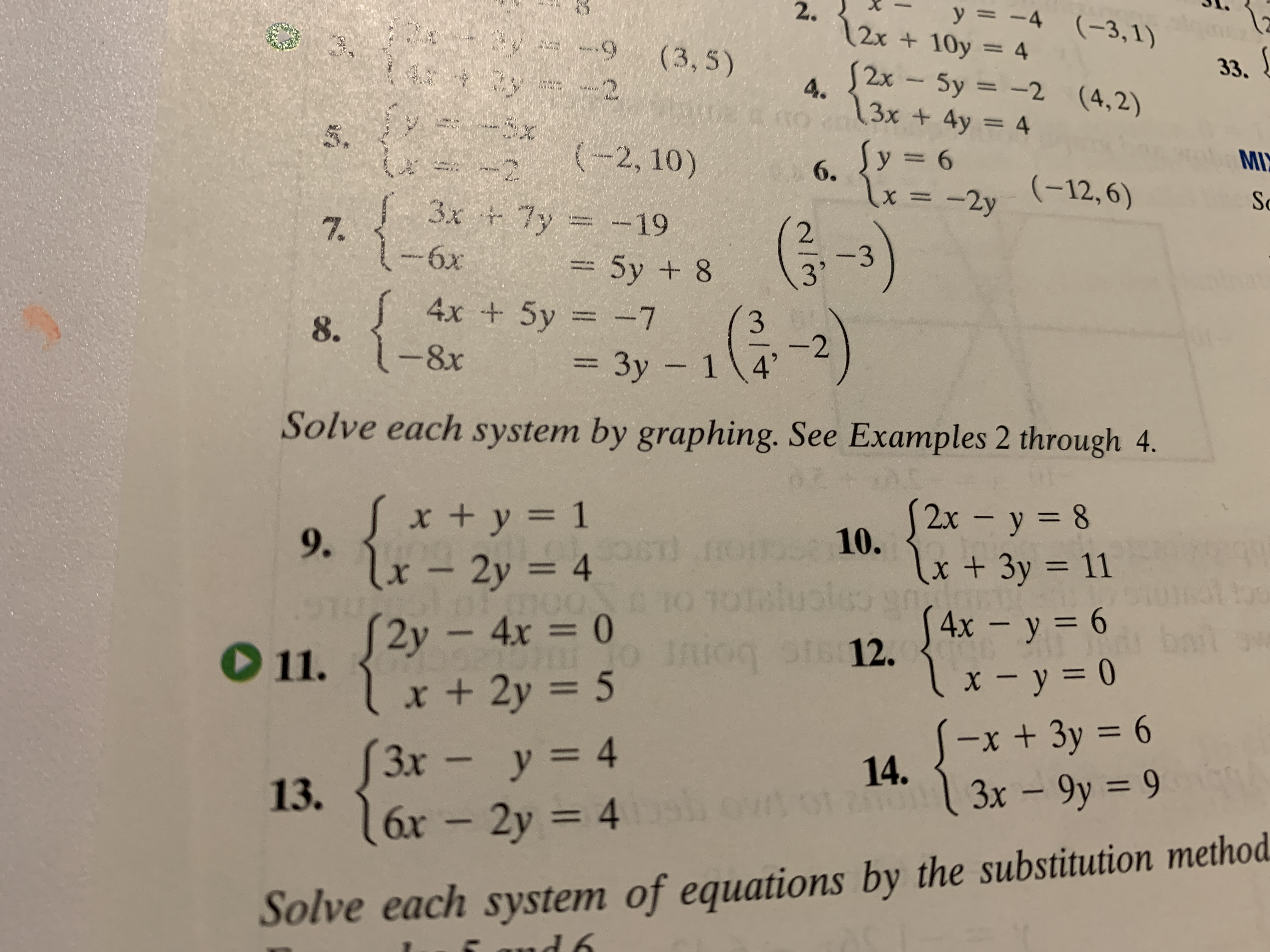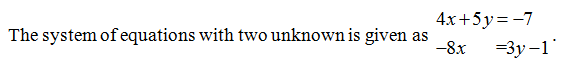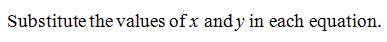# y = -4 (-3,1)2.12x +10y 4(3, 5)233.S2xSy =-2 (4,2)4.3x + 4y = 4(-2, 10)y 6MI-26.lx =(-12, 6)-2ySc3x 7y-197.2-33'-6x5y +84x + 5y = -7{3-2Зу - 1 4°8.- 8x= 3y -Solve each system by graphing. See Examples 2 through 4.x + y = 19.2x- y 810.x+ 3y 11n1otslusleoSTS12.lx - 2y = 4AIN0 TOS4x - y 6lx-y= 0-x + 3y 63x - 9y 92y - 4x = 0x + 2y 5bat11.3x-y = 413.16x- 2y 414.120ЗхSolve each system of equations by the substitution methodd 6il

Question
51 viewshelp_outlineImage Transcriptionclosey = -4 (-3,1) 2. 12x +10y 4 (3, 5) 2 33. S2x Sy =-2 (4,2) 4. 3x + 4y = 4 (-2, 10) y 6 MI -2 6. lx = (-12, 6) -2y Sc 3x 7y -19 7. 2 -3 3' -6x 5y +8 4x + 5y = -7 { 3 -2 Зу - 1 4° 8. - 8x = 3y - Solve each system by graphing. See Examples 2 through 4. x + y = 1 9. 2x- y 8 10. x+ 3y 11 n 1otslusleo STS12. lx - 2y = 4 AIN 0 TO S4x - y 6 lx-y= 0 -x + 3y 6 3x - 9y 9 2y - 4x = 0 x + 2y 5 bat 11. 3x-y = 4 13. 16x- 2y 4 14. 120 Зх Solve each system of equations by the substitution method d 6 il fullscreen
check_circle

Step 1Step 2...

### Want to see the full answer?

See Solution

#### Want to see this answer and more?

Solutions are written by subject experts who are available 24/7. Questions are typically answered within 1 hour.*

See Solution
*Response times may vary by subject and question.
Tagged in

### Algebra